# Get these flashcards, study & pass exams. For free! Even on iPhone/Android!

## Algebra 1 (10 Cards)

Theorem 0.1 Division Algorithm
Let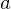and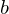be integers with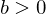. Then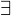unique integers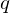and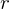with the property that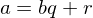, where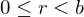.
Theorem 0.2 GCD is a Linear Combination
For any nonzero integersand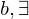integers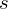and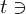gcd(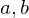)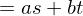. Moreover, gcd() is the smallest positive integer of the form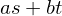.
Euclid's Lemma
if p is prime then p|ab implies p|a and/or p|b.
Theorem 0.3 Fundamental Theorem of Arithmetic
Every integer greater than 1 is a prime or the unique product of primes.
Theorem 0.4 First Principle of Mathematical Induction
Let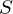be a set of integers containing. Supposehas the property that whenever some integer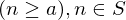, then the integer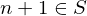. Then,contains every integer greater than or equal to.
Theorem 0.5 Second Principle of Mathematical Induction
Letbe a set of integers containing. Supposehas the property that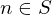whenever every integer less than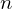and greater than or equal tobelongs to. Then,contains every integer greater than or equal toEquivalence Relation
An equivelence relation on a set S is a set R of ordered pairs ot elements of S such that:
(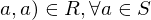(reflexive)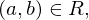implies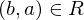(symmetric)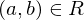and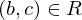imply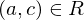(transitive)
Partition
A partition of a setis a collection of nonempty disjoint subsets ofwhose unions is.
Theorem 0.6 Equivalence Classes Partition
The equivalence classes of an equivalence relation on a setconstitute a partition of. Conversely, for any partition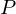of, there is an equivalence relation onwhose equivalence classes are the elements ofFunction (Mapping)
A function (or mapping)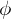from a set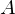to a set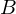is a rule that assigns to each elementofexactly one elementof. The setis called the domain ofandis called the range of. Ifassignsto, thenis called the image ofunder. The subset ofcomprising all the images of elements ofis called the image of A under.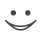Flashcard set info:
Author: Squiggleart
Main topic: Mathematics
Topic: Abstract Algebra
School / Univ.: West Chester University
Published: 25.05.2011
Tags: Algebra

Card tags:
All cards (10)
no tagsReport abuse